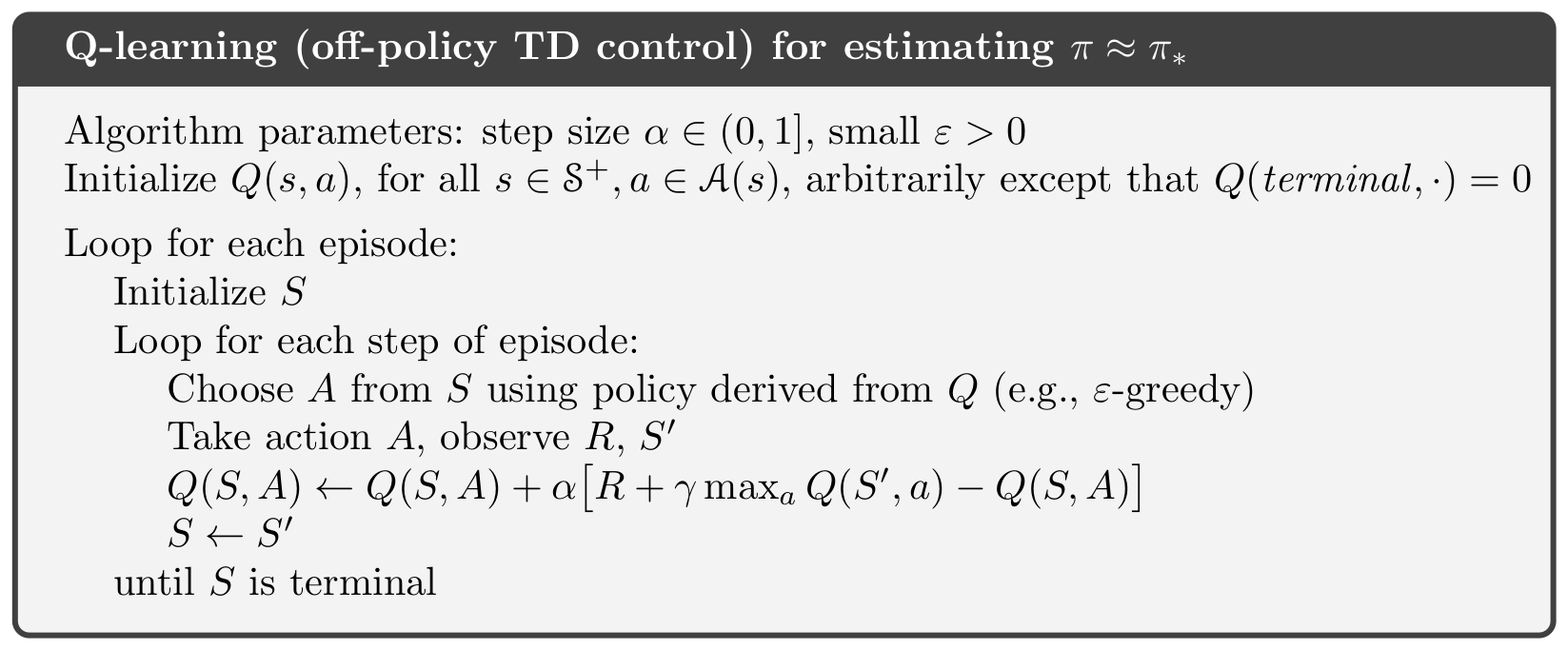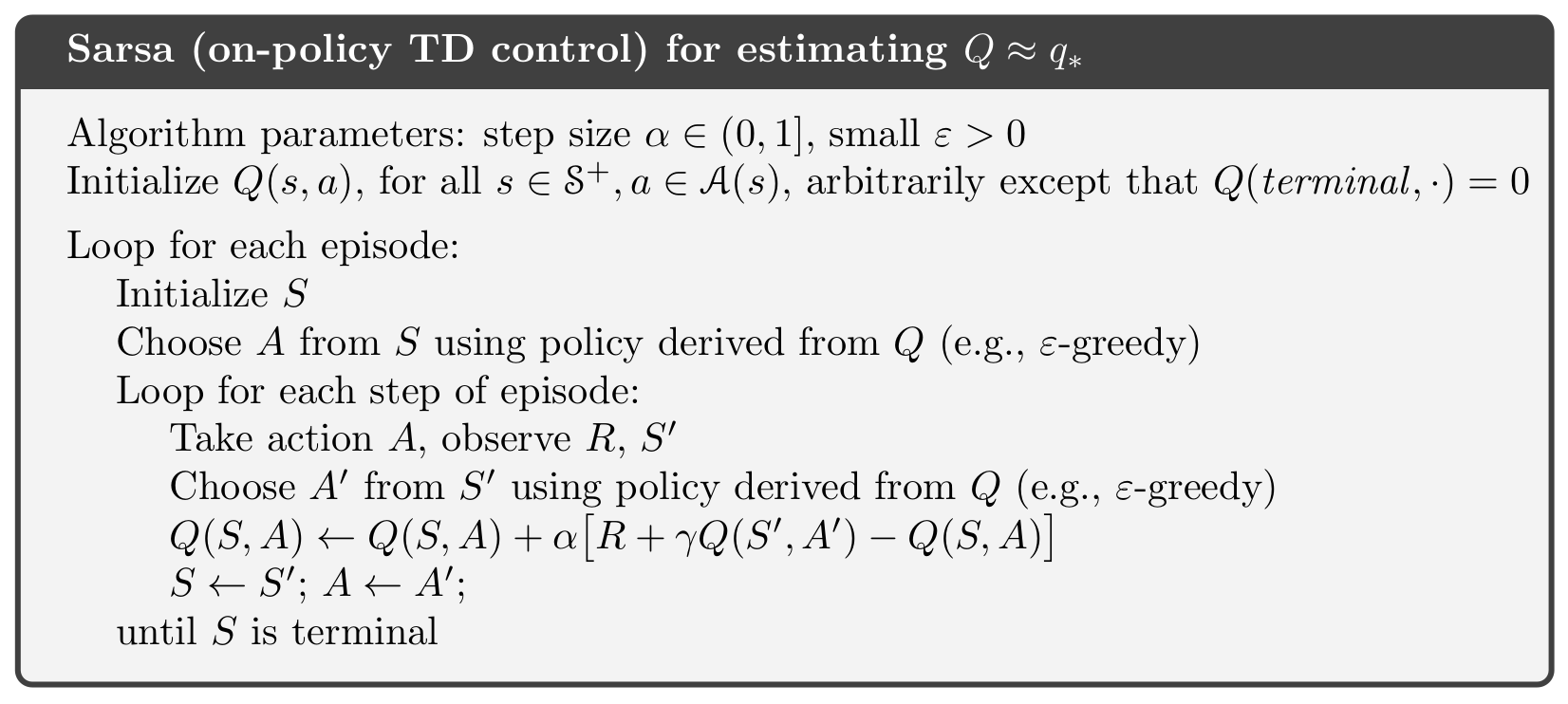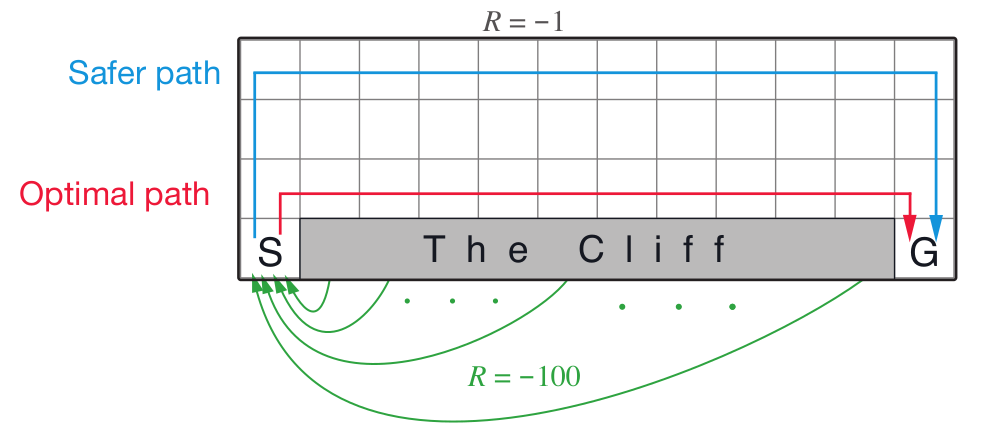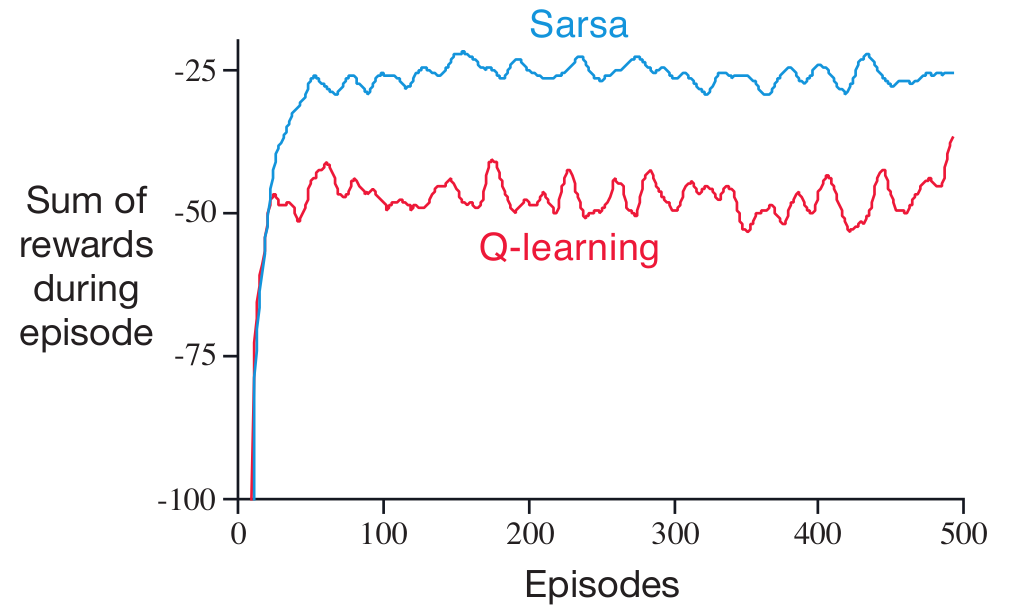# On-Policy VS Off-Policy in Reinforcement Learning

## Introduction

I have not been working on reinforcement learning for a while, and it seems that I could not remember what on-policy and off-policy mean in reinforcement learning and what the differences between these two are. Most of explanations online bluff too much and I don’t think those are directly answering the questions.

I also do have to apologize that I have taken several good images from Sutton’s latest book “Reinforcement Learning: An Introduction” 2nd Edition.

## Algorithms

Let us review a typical off-policy algorithm Q-Learning and a typical on-policy algorithm SARSA first.

### Q-Learning### SARSA## Off-Policy VS On-Policy

We can see that the major difference is that Q-Learning is using

$$Q(S,A) \leftarrow Q(S,A) + \alpha [R + \gamma \text{max}_{a} Q(S’,a) - Q(S,A)]$$

to update Q value. While SARSA is using

$$Q(S,A) \leftarrow Q(S,A) + \alpha [R + \gamma Q(S’,A’) - Q(S,A)]$$

to update Q value.

Let us introduce two concepts first: update policy and behavior policy.

Update policy is how your agent learns the optimal policy, and behavior policy is how your behaves.

In Q-Learning, the agent learns optimal policy using absolute greedy policy and behaves using other policies such as $\varepsilon$-greedy policy. Because the update policy is different from the behavior policy, so Q-Learning is off-policy.

In SARSA, the agent learns optimal policy and behaves using the same policy such as $\varepsilon$-greedy policy. Because the update policy is the same as the behavior policy, so SARSA is on-policy.

## Cliff Walking Game

I took the Cliff Walking game from Sutton’s book. It seems that Q-Learning with $\varepsilon$-greedy action learns the value for optimal policy but sometimes it will fall off due to $\varepsilon$-greedy action. SARSA with $\varepsilon$-greedy action learns the value for a less optimal policy but it is a safer policy.To me, it seems that Q-Learning with $\varepsilon$-greedy action will be unstable (less likely to converge) during learning in some environments but it is more likely to learn an optimal policy as the overall reward is lower and fluctuation is larger. SARSA, on the other hand, will be more stable during learning but it is less likely to learn an optimal policy.According to Sutton, “if $\varepsilon$ were gradually reduced, then both methods would asymptotically converge to the optimal policy.”

## Final Remarks

I actually have not seen any formal definition about on-policy and off-policy from authority publications (Who invented these?). What if I modified Q-Learning algorithm to use absolute greedy policy to behave, is this new algorithm on-policy now?

On-Policy VS Off-Policy in Reinforcement Learning

https://leimao.github.io/blog/RL-On-Policy-VS-Off-Policy/

Lei Mao

03-14-2019

03-14-2019